Hans Walser, 

Sphere and cylinder

# 1     The original problem

In (Richeson 2017, p 23) I found:

“Just noticed that the ratio of the volume of a sphere to the volume of the cylinder containing it is 2:3.

Likewise, the surface area. Eureka!”Fir. 1: Sphere and cylinder

# 2     In other dimensions

The mentioned statement holds similar in other dimensions.

We use the following notations: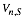= Volume of the sphere in the n-dimensional space= Surface of the sphere in the n-dimensional space= Volume of the cylinder in the n-dimensional space= Surface of the cylinder in the n-dimensional space

Examples: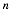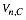Ratio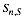Ratio 2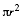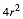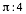3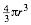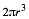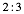4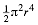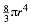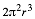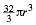5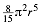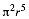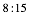6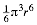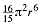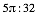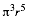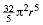7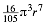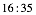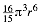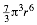Tab. 1: Examples

In every dimension there is the same ratio. In even dimensions the ratio is irrational.

# 3     General case

Notation:(1)

Hence we get:(2)

And for the volume of the cylinder: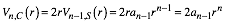(3)

For the surface of the cylinder we get:(4)

Remark:(5)

The volumes of the sphere and the cylinder have the ratio:(6)

For the surfaces we get the ratio: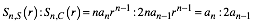(7)

From (6) and (7) we see that the ratios are equal in any dimension.

# 4     Explicit formulas

According to  we have:(8)

From (6) and (8) we get:

In even dimensions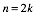we have:

ratio =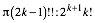(9)

!! denotes the double faktorial, defined for odd integersby:(10)

In odd dimensions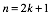we get:

ratio =(11)

References

Richeson, David (2017): A-Tweeting We Will Go. Building a Professional Network with Twitter. MAA FOCUS | JUNE/JULY 2017 | maa.org/focus. 22-25.

Websites

 Wikipedia: n-sphere

https://en.wikipedia.org/wiki/N-sphere#Volume_and_surface_area